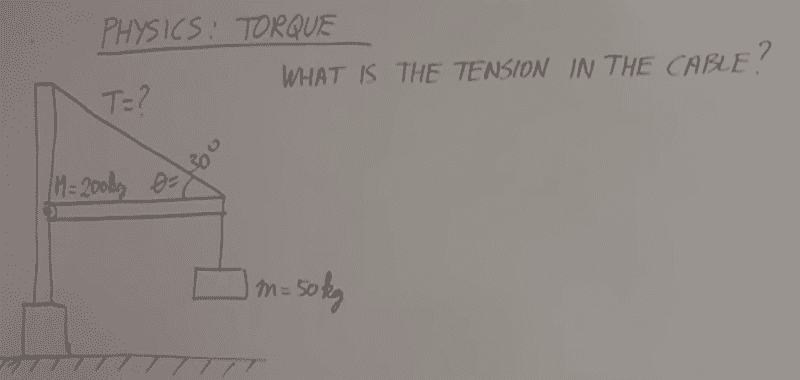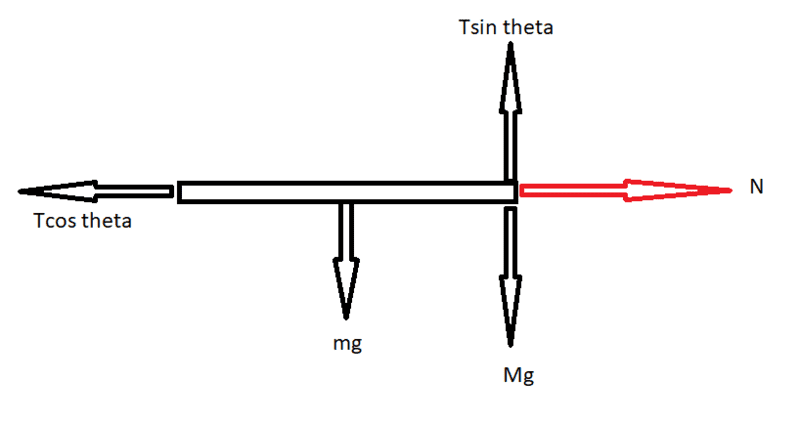# Static equilibrium: Find the tension in a support cable

## Homework Statement## Homework Equations

Σtorque = 0
Σfx=0
Σfy=0
torque= F r sinθ (θ is the angle between the r and the F)

## The Attempt at a Solution

OKay, now i know how to solve this, but it's like i asked myself, how to know if this is a question that i am supposed to use
Σfx=0 and Σfy=0 (Newton's law lesson) and get the answer or to use the Σtorque=0 with Σfx=0, Σfy=0. so i said let me solve this question as if i still didn't take the torque yet, and if i couldn't reach to any answer and have not enough known values , then of course i will have to use the sum of torque= 0 to reach the answer. ( Know that this question can be solved only by the sum of torque=0 without going through the sum of forces).
so i started to find the Σfx=0 forces
and couldn't find only one force which is the x component of the tension force ( Tcos(30) to the west) and what about the other force to the right? is it the N force from the wall or what.
i said maybe it's N force ( not sure), and it's not written here since the torque of this force will be zero because it passes through the pivot point chosen (it's shown near the wall in the figure). so i want to know what is the opposite x force to the east to cancel Tcos(30).
and for y it would be: Σfy=0
Tsin30 = Mg + mg
right?

#### Attachments

Orodruin
Staff Emeritus
Homework Helper
Gold Member
2021 Award
and couldn't find only one force which is the x component of the tension force ( Tcos(30) to the west) and what about the other force to the right? is it the N force from the wall or what.
This depends on what parts of the structure you are including in your free-body diagram. Without specifying that, you cannot make force equations or torque equations.

and for y it would be: Σfy=0
Tsin30 = Mg + mg
right?
Does it match the result you get for ##T## if you only consider the torque. If not, then it is not correct.

•SakuRERE
This depends on what parts of the structure you are including in your free-body diagram. Without specifying that, you cannot make force equations or torque equations.
yeaah, i got what you mean. i am taking the hanged plate as the body being balanced so :i computed T from the Sum of torque and got T equal to 2943 N which is definitely correct. and so i applied here
sum of fy=0 and found T as if it's unknown and got T= 4905 N which means it's not right.sum of fy=0
Tsin theta -mg -Mg=0 ......
T=4905 N

#### Attachments

then it is not correct.
then how do i know the opposite force ?

Orodruin
Staff Emeritus
Homework Helper
Gold Member
2021 Award
First of all, the horizontal forces that you have drawn do not have the correct point of application.

and so i applied here
sum of fy=0 and found T as if it's unknown and got T= 4905 N which means it's not right.
So this means that you are making a faulty assumption somewhere. Your torque analysis around the pivot point is correct, so this means that you are missing a force or a force component that has zero torque relative to the pivot point in your force equilibrium equations.

•SakuRERE
First of all, the horizontal forces that you have drawn do not have the correct point of application.

So this means that you are making a faulty assumption somewhere. Your torque analysis around the pivot point is correct, so this means that you are missing a force or a force component that has zero torque relative to the pivot point in your force equilibrium equations.
yeaah, It's not easy as i thought.
it's not even right to say :
Tsin30 = Mg-mg+F (F is the no known force)
and i know that T= 2943 N from the sum of torque
so trying to find F i get :
F= -981 N
does this indicates that the force is up or down. i assumed first that it would be down. since i got first 4905N when it should be 2943 N . so of course the force direction i am trying to find is down to decrease that 4905 N. So, F=981N is downward. and imagining this is a weight for something then it would have a mass of (100kg) but there is nothing that could have this force. i am confused, but at the same time, i really want to solve it

Orodruin
Staff Emeritus